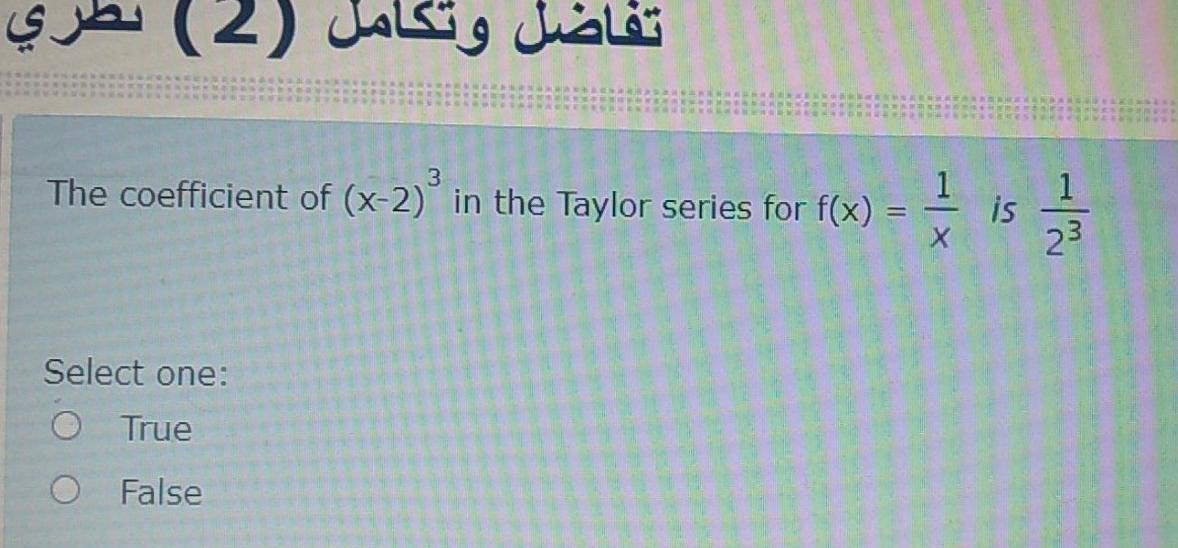### Create an Account

Home / Questions / تفاضل وتكامل (2) The coefficient of (x-2) in the Taylor series for f(x) = 1 1 is 23 Select...

# تفاضل وتكامل (2) The coefficient of (x-2) in the Taylor series for f(x) = 1 1 is 23 Select one: O True O False

تفاضل وتكامل (2) The coefficient of (x-2) in the Taylor series for f(x) = 1 1 is 23 Select one: O True O FalseApr 26 2021 View more View LessSubscribe To Get Solution